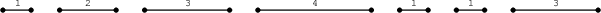Your browser (Unknown 0) is no longer supported. Some parts of the website may not work correctly. Please update your browser.

#### TieRopes

Tie adjacent ropes to achieve the maximum number of ropes of length >= K.

There are N ropes numbered from 0 to N − 1, whose lengths are given in an array A, lying on the floor in a line. For each I (0 ≤ I < N), the length of rope I on the line is A[I].

We say that two ropes I and I + 1 are adjacent. Two adjacent ropes can be tied together with a knot, and the length of the tied rope is the sum of lengths of both ropes. The resulting new rope can then be tied again.

For a given integer K, the goal is to tie the ropes in such a way that the number of ropes whose length is greater than or equal to K is maximal.

For example, consider K = 4 and array A such that:

A = 1 A = 2 A = 3 A = 4 A = 1 A = 1 A = 3

The ropes are shown in the figure below.We can tie:

• rope 1 with rope 2 to produce a rope of length A + A = 5;
• rope 4 with rope 5 with rope 6 to produce a rope of length A + A + A = 5.

After that, there will be three ropes whose lengths are greater than or equal to K = 4. It is not possible to produce four such ropes.

Write a function:

class Solution { public int solution(int K, int[] A); }

that, given an integer K and a non-empty array A of N integers, returns the maximum number of ropes of length greater than or equal to K that can be created.

For example, given K = 4 and array A such that:

A = 1 A = 2 A = 3 A = 4 A = 1 A = 1 A = 3

the function should return 3, as explained above.

Write an efficient algorithm for the following assumptions:

• N is an integer within the range [1..100,000];
• K is an integer within the range [1..1,000,000,000];
• each element of array A is an integer within the range [1..1,000,000,000].